### 15.4.3.4 Controllability of driftless systems

The controllability of a driftless control-affine system (15.53) can be characterized using the Lie algebra rank condition (or LARC). Recall the definition of STLC from Section 15.1.3. Assume that either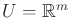or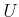at least contains an open set that contains the origin of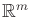. The Chow-Rashevskii theorem [112,156,846] states:

A driftless control-affine system, (15.53), is small-time locally controllable (STLC) at a point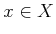if and only if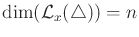, the dimension of.

If the condition holds for every, then the whole system is STLC. Integrability can also be expressed in terms of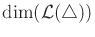. Assume as usual that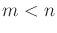. The three cases are: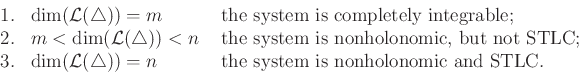(15.110)

Example 15..19 (Controllability Examples)   The differential drive, nonholonomic integrator, and the system from Example 15.17 are all STLC by the Chow-Rashevskii theorem because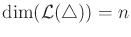. This implies that the state can be changed in any direction, even though there are differential constraints. The state can be made to follow arbitrarily close to any smooth curve in. A method that achieves this based on the Lie algebra is given in Section 15.5.1. The fact that these systems are STLC assures the existence of an LPM that satisfies the topological property of Section 14.6.2.Steven M LaValle 2020-08-14